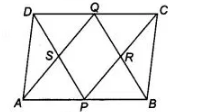# P and Q are the mid-points of the opposite`
Question:

P and Q are the mid-points of the opposite sides AB and CD of a parallelogram ABCD. AQ intersects DP at S and BQ intersects CP at R. Show that PRQS is a parallelogram.

Solution:

Given In a parallelogram ABCD, P and Q are the mid-points of AS and CD, respectively.

To show PRQS is a parallelogram.

Proof Since, ABCD is a parallelogram.

AB||CD

=> AP || QC

Also, $A B=D C$$\frac{1}{2} A B=\frac{1}{2} D C$   [dividing both sides by 2$]$

$\Rightarrow \quad A P=Q C$ [since, $P$ and $Q$ are the mid-points of $A B$ and $D C$ ]

Now, $A P \| Q C$ and $A P=Q C$

Thus $A P C Q$ is a parallelogram.

$\therefore \quad A Q \| P C$ or $S Q \| P R \quad \ldots$ (i)

Again,   $A B \| D C$ or $B P \| D Q$

Also, $A B=D C \Rightarrow \frac{1}{2} A B=\frac{1}{2} D C$ [dividing both sides by 2]

$\Rightarrow \quad B P=Q D \quad$ [since, $P$ and $Q$ are the mid-points of $A B$ and $D C$ ]

Now,  $B P \| Q D$ and $B P=Q D$

So, $B P D Q$ is a parallelogram.

$\therefore \quad P D \| B Q$ or $P S \| Q R$ ... (ii)

From Eqs. (i) and (ii), $S Q \| R P$ and $P S \| Q R$

So, PRQS is a parallelogram. Hence proved.